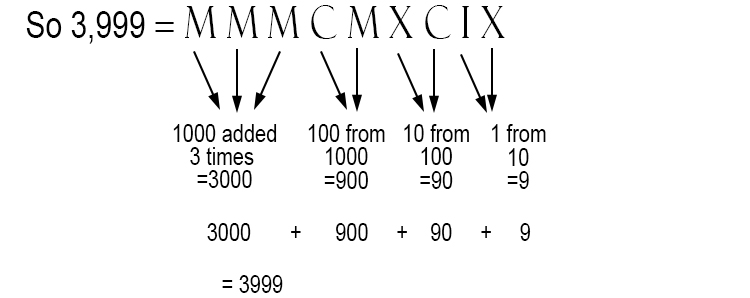# Roman numerals greater than 1000

The rule for Roman numerals is that you cannot use more than three of the same symbol in a row. We know now that the way to remember the symbols is by remembering:

'I Value Xylophones Like Cows Do Milk'.
I=1,  V=5,  X=10,  L=50,  C=100,  D=500,  M=1000But 4000 would then  = MMMM and you can't have more than three of the same symbol in a row.

The Romans got over this by putting a dash over any symbol to represent 1000 times the value of that symbol.

(Also note that Romans sometimes used an M and sometimes bar I but they mean the same thing).

I = 1

V = 5

X = 10

L = 50

C = 100

D = 500

M = 1000

bar I = 1000 x 1 = 1000

bar V = 1000 x 5 = 5,000

bar X = 1000 x 10 = 10,000

bar L = 1000 x 50 = 50,000

bar C = 1000 x 100 = 100,000

bar D = 1000 x 500 = 500,000

bar M = 1000 x 1000 = 1 million

The number 4000 thus becomes:

4000 = Mbar V

4001 = Mbar VI

4002 = Mbar VII

4003 = Mbar VIII

4004 = Mbar VIV